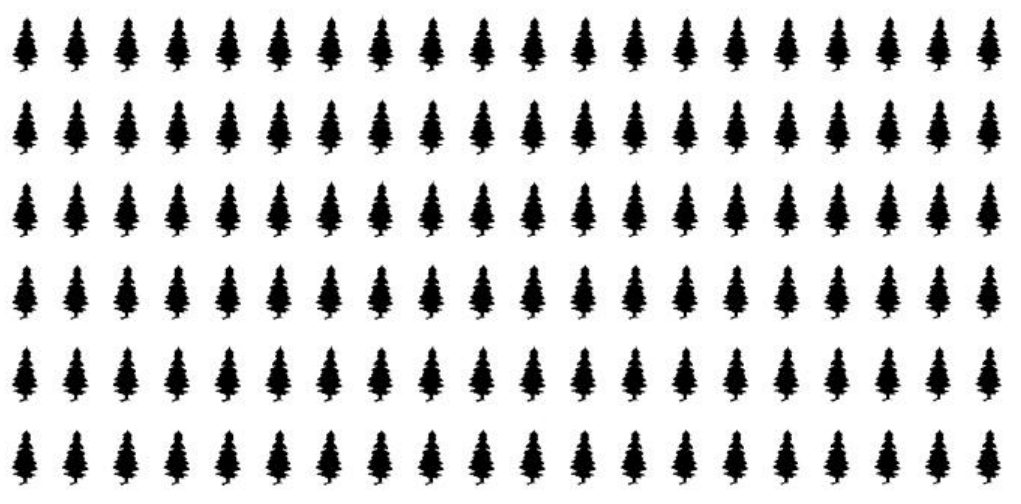Subject:
Mathematics
Problem Solving

Topics: Problem SolvingRatios
Common Core State Standard: 6.RP.3,
Concepts:

· Ratio

Knowledge and Skills:

· Can solve complex, multi-step problems
· Understands and can use three methods of expressing a ratio (“2 to 3”, “2:3”, “2/3”)
· Can use proportional reasoning to solve problems

Lesson:

Procedure: This activity can be done by students individually or in teams of two.

Hand out the activity sheet to each student or team. Ask a student to read aloud from the sheet, up to the point where it says that the ranger has 20 ponderosa pines.

Ask students to explain what is mean by the statement that “Ponderosa pine: Douglas fir = 2:1”, and to give you examples of numbers of trees that would make this statement true. In this discussion, review the meaning of “ratio” and the ways that ratios can be written.

Ask students to work on their own (or in their teams) to answer questions #1, 2, and 3. Circulate as they do so and give help as needed. (Answers: 10 Douglas fir trees, 30 cedar trees, total of 60 trees.)

Once all students have completed this part of the activity, ask a few students to present their answers and explain how they got those answers.

Ask students to work on their own (or in their teams) to answer questions 4, 5, and 6. Circulate as they do so, and guide them as needed.

One way to use the diagram is to mark one tree as a Douglas Fir (by coloring it red, say). According to the ratios, there would then need to be two Ponderosa Pines marked (in a different color) , and three cedars (in a different color). This process can be repeated until all of the trees are “used up”.  An advantage of this approach is that it is likely that students would soon start marking more than one Douglas fir at a time (and correspondingly more of the others trees), as they realize that the numbers of trees marked at one time can be doubled, tripled etc. as long as the ratios remain the same.

### The Forest and the Trees

Forest ranger Arnold Wilson wants to plant three kinds of trees on a mountain: Ponderosa Pine, Douglas fir, and White Fir.

He has decided that he wants these ratios for the trees:

Ponderosa Pine: Douglas Fir = 2:1

White Pine: Ponderosa Pine = 3:2

Suppose he has 20 Ponderosa Pine trees to plant. Help him by answering these questions:

1. How many Douglas Fir trees should he plant?

2. How many White Fir trees should he plant?

3. How many trees total would be plant?

Suppose he decides he wants to plant a total of 120 trees.

4. How many Ponderosa Pine trees should he plant?

5. How many Douglas Fir trees should he plant?

6. How many White Fir trees should he plant?